# Introduction to Digital Logic Gates

What is digital logic and how do logic gates work?

## Circuit with Single Switch and Truth Table

Let us examine the two basic circuits below. The top one has an open switch and therefore the bulb is OFF. The second one is closed and the bulb is ON.

The bulb in ON or OFF depending on the position of the switch. Let use 0 to describe the switch when it is open and 1 when the switch is closed. We also use 0 to describe the bulb when it is OFF and 1 when the bulb is ON to write all possible situations in the following table.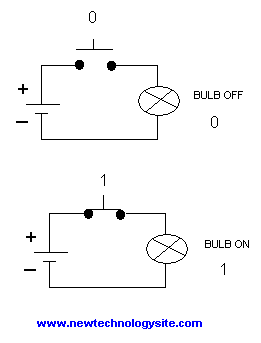Fig. 1 - Single Switch

 Input (switch) Output (Bulb) 0 0 1 1

## Circuit with Two Switches in Parallel and Truth Table

We now examine circuits with two switches in parallel as in the figure below. Since the switches are in parallel, if either switch X is closed or switch Y is closed or both are closed the circuit is closed and the bulb is ON. The table below includes all possible combinations of the positions of the two switches and the corresponding state of the bulb (ON or OFF).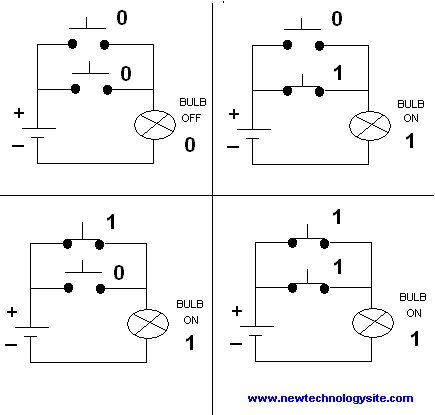Fig. 2 - Circuit with Two Switches in Parallel

 Top Switch: X Bottom Switch: Y Output (bulb) 0 0 0 0 1 1 1 0 1 1 1 1

The above table corresponds exactly to the OR function as defined in Boolean algebra.

Using Boolean algebra, the results in the table above may be written as follows:

 X Y X+Y 0 0 1 0 1 1 1 0 1 1 1 1

Although the first three sums have the same meaning as in any mathematical addition, the last sum 1 + 1 = 1 suggests that the meaning of addition here is different. This is boolean algebra which originally used "TRUE" and "FALSE" before it was adopted in digital systems and 1 and 0 replaced the "TRUE" and "FALSE".

## Circuit with Two Switches in Series and Truth Table

We now examine circuits with two switches in series as in the figure below. Since the switches are in series, the bulb is ON only when both switches are closed. The table below includes all possible combinations of the positions of the two switches and the corresponding state of the bulb (ON or OFF).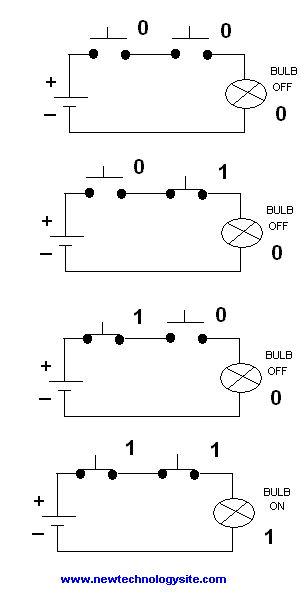Fig. 3 - Circuit with Two Switches in Series

 Left Switch: X Right Switch: Y Output (bulb) 0 0 0 0 1 0 1 0 0 1 1 1

The above table corresponds exactly to the AND function as defined in Boolean algebra.

Using Boolean algebra, the results in the table above may be written as follows:

 X Y X . Y 0 0 0 0 1 0 1 0 0 1 1 1

This is boolean algebra which originally used "TRUE" and "FALSE" before it was adopted in digital systems and 1 and 0 replaced the "TRUE" and "FALSE".

## AND and OR Digital Circuits and Digital Logic

The above circuits with switches were used to explain the idea behind digital logic circuits. In practice these circuits are electronic circuits and their representation is as follows: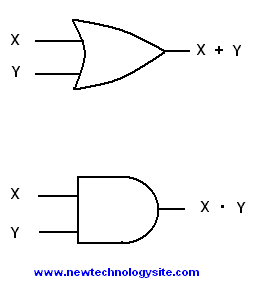Fig. 4 - OR and AND logic gates

## XOR Digital Circuit and Digital Logic

The logic gate exclusive OR (XOR) is defined by its table of input and output values as follows: .

 X Y X ⊕Y 0 0 0 0 1 1 1 0 1 1 1 0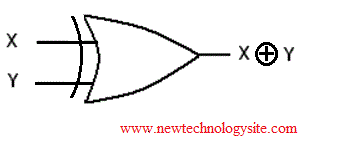Fig. 5 - XOR logic gates